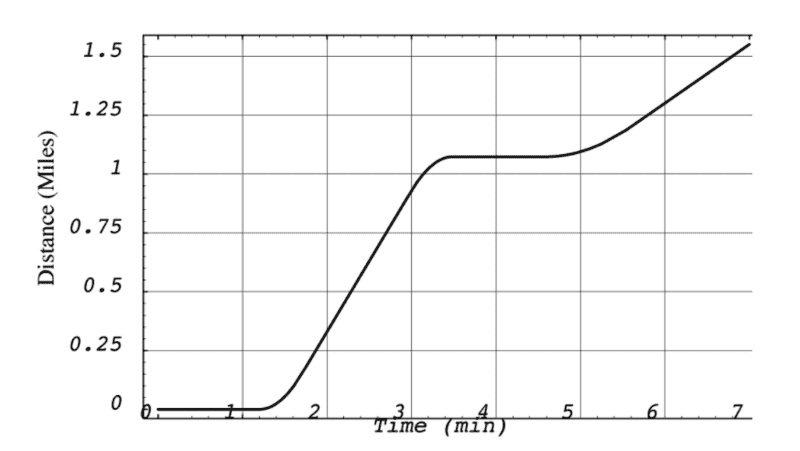# Acceleration and speed -- confused

mncyapntsi
Homework Statement:
Which of the following statements are false:

The speed at 2.1 min is less than at 2.6 min.
The acceleration is zero at 0.5 min and 4.2 min.
The acceleration is non-zero at 6.2 min.
The acceleration is never negative.
The acceleration is non-zero at 2.3 min.

IMAGE BELOW
Relevant Equations:
a(t) = dv/dt; v(t) = dx/dt; ...
I've written in that only the first statement was False, however my classmates aren't getting the same answer?Homework Helper
Gold Member
2022 Award
Can you explain why you think the rest are true?

mncyapntsi
Can you explain why you think the rest are true?
Here is my reasoning:

The acceleration is zero at 0.5 min and 4.2 min: true because x(t)=constant so v(t)=0 so a(t)=0

i just rethought this one - false right?: The acceleration is non-zero at 6.2 min: false because x(t)=mx+p so v(t)=constant so a(t)=0

The acceleration is never negative: true bc nowhere is v(t)<0

i just rethought this one - false? The acceleration is non-zero at 2.3 min: false because x(t)=mx+p so v(t)=constant so a(t)=0

•PeroK
Homework Helper
Gold Member
2022 Award
The acceleration is never negative: true bc nowhere is v(t)<0
Negative acceleration means that the object is slowing down. Not that the velocity is negative.

•mncyapntsi
mncyapntsi
Right! So only one of them is true - the second one?

Homework Helper
Gold Member
2022 Award
Right! So only one of them is true - the second one?
That's what I think.

•mncyapntsi
mncyapntsi
Thanks for helping me think through that, and clearing it up!•PeroK
•